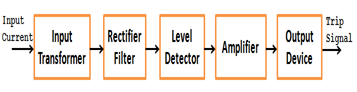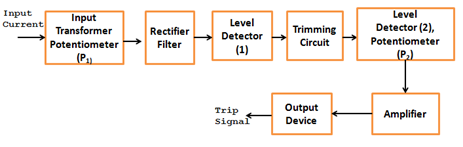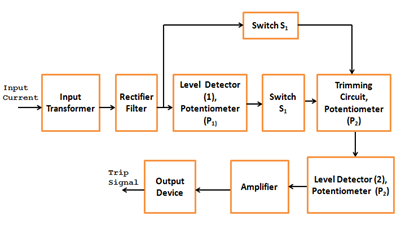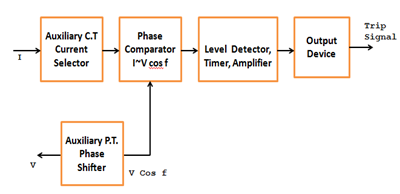# Instantaneous, Definite Time, Inverse-Time OverCurrent RelaysStatic relays are mainly made with semiconductor switches like thyristor. In digital relays there are two states i.e., ON and OFF which can be referred to 1 and 0 state. Based on Characteristics some the protection relays are detailed below:
1. Instantaneous overcurrent relays.
2. Definite time overcurrent relays
3. Inverse-time overcurrent relays with definite minimum time (IDMT)
4. Directional overcurrent relays

# Instantaneous Overcurrent RelaysThe block schematic diagram of the static instantaneous Overcurrent relay is shown in the figure. The current derived from the CT is fed to the input transformer which gives a proportional output voltage. The input transformer has an air gap in the iron core to give linearity in the current / voltage relationship up to the highest value of current expected and is provided with tapping on its secondary winding to obtain different current settings. The output voltage of the transformer is rectified through a rectifier and then filtered at a single stage to avoid undesirable time delay in filtering, so as to ensure high speed of operation. A limit made of a zener diode is also incorporated in the circuit to limit the rectified voltage to safe values even when the input current is very high under fault conditions. A fixed portion of the rectified and filtered voltage (through a potential divider) is compared against a preset pick-up value by a level detector and if it exceeds the pick-up value, a signal through an amplifier is given to the output which issues the trip signal. The output device may either be a static thyristor circuit or an electromagnetic slave relay.

## Definite Time Overcurrent Relays

The operating time of overcurrent relays are constant irrespective of the level of the fault current. In this case, an intentional time delay is introduced through a timing circuit. The figure below shows that the simplified block diagram of a definite time overcurrent relay. The input current signal derived from the main CT is converted to a proportional voltage signal by the input transformer and then rectified, filtered and compared with the preset threshold value of the level detector (1). If the voltage exceeds the preset threshold value, the level detector gives an output voltage, thereby the charging of the capacitor C of the RC timing circuit starts. As soon as the voltage across the capacitor exceeds the preset threshold value (VT) of level detector (2), a signal through the amplifier is given and time setting, respectively.If VT is the threshold value of the level detector, the time TC required to reach this voltage depends up,on the charging time of the capacitor C of the RC timing circuit, given by,

TC = RCloge [V / (V-VT)]

Where V is the voltage applied to the capacitor. If V, R and C are constant, the charging time for a given value of VT will be constant. The time TC can be varied by varying R-C combinations and VT. In this case, since the capacitor charging is done from a fixed d.c. output, voltage of level detector (1), the operating time of the relay for particular values of R and C of the timing circuit and VT of the level detector (2) will be constant for different values of the fault current.

### Inverse-Time Overcurrent Relay

The operating time of the inverse-time overcurrent relays decreases with increasing fault current. For this relay with inverse-time characteristic, the charging of the capacitor of the timing circuit takes place from a voltage proportional to current.The block diagram of the inverse-time overcurrent relay is shown in the figure. The current signal is converted to a proportional voltage signal by the input transformer and then rectified, filtered and compared with a reference voltage of the level detector (1) set by the potentiometer P1. Under normal conditions i.e., when the input current is low switch S1 is ON, short circuiting the capacitor C of the RC timing circuit and switch S2 is OFF. As soon as the input voltage exceeds the preset reference voltage of the level detector (1), switch S1 is switched OFF and switch S2 is switched ON and the charging of capacitor C of the timing circuits starts from a voltage proportional to the current. Switches S1 and S2 are made of static components. When the voltage across the capacitor C of the timing circuit exceeds the reference voltage of the level detector (2) as set by potentiometer P3, a signal is given to the output device through an amplifier. Finally, the output device issues the trip signal. Here the plug setting multiplier is given by the transformer secondary tap and potentiometer P1 and the time multiplier setting is determined by potentiometers P2 and P3.

### Directional Overcurrent Relays

The directional overcurrent relays incorporates a directional unit which responds to power flow in a specified direction. The directional relay senses the direction of power flow by means of a phase difference (Φ) between voltage (V) and current (I). When Φ exceeds a certain relay operates. The directional relay is a double actuating quantity relay with one input as current I from CT and the other input as voltage V from PT.

In case of electromagnetic directional overcurrent relays, discrimination is affected when voltage drops down to very low values under fault conditions. In static directional relays. this problem is less serious because the static comparators used in these relays are inherently very sensitive and they can give reliable performance up to 1% of system voltage which is well within the minimum fault voltage.The above figure shows that the simplified block diagram of the directional overcurrent relay. The inputs V and I are applied to phase comparator. A phase shifter is added in voltage input circuit before applying it to the phase comparator to achieve the maximum output of the phase comparator under fault conditions. The output of the phase comparator is given to the level detector and then to the output device through an amplifier. if the output of the phase comparator exceeds the preset reference voltage of the level detector, the output device issues the trip signal.

There are two main types of phase comparators used for the purpose. One of these is the Hall effect type comparator which has been used in USSR, whereas all other countries have preferred the rectifier bridge type of comparator due to its lower cost and the higher output obtainable as compared to the Hall elements.

This post first appeared on My Tech Info, please read the originial post: here

# Share the post

Instantaneous, Definite Time, Inverse-Time OverCurrent Relays

×